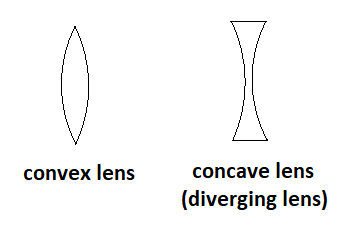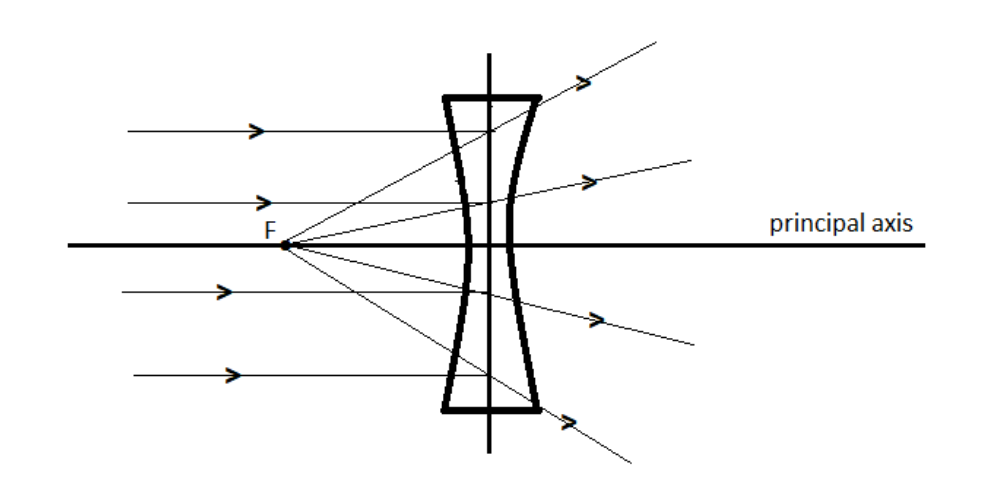Courses
Courses for Kids
Free study material
Free LIVE classes
MoreLIVE
Join Vedantu’s FREE Mastercalss

# Which lens is known as a diverging lens?A. Convex.B. Concave.C. Spherical.D. Plano convex.Verified
346.8k+ views
Hint: We have to know about the working of lenses to answer this problem and we should know about a diverging lens . A lens positioned in a parallel radiation beam's direction may be called a diverging lens as it causes the rays to diverge after refraction.

Complete Step-by-Step solution:
The working of a lens is dependent on light rays refraction as they pass through it.
A lens is a transparent piece of glass connected by two spherical surfaces.
(I) The convex lens at the middle is thicker but the edges thinner.
(ii) The concave lens in the middle is thin but the sides are thicker.
We recognize that a diverging lens diverges a light beam, and it generates a composite image for all object positions.As light rays penetrate a concave lens, they diverge, making the lens act like a diverging lens. And when working in the typical climate, a concave lens may be called a diverging lens.
Hence, we can say that a concave lens is also known as a diverging lens.
Therefore, the correct answer is option (B).

Note: We should realize that the lenses diverging and the lenses converging are two different lenses with different properties, not the same ones. A converging lens is a lens which converges light rays which travel parallel to its main axis. Their upper and lower edges are moderately thick around their middle and thin. A diverging lens is a lens which diverges light rays and moves parallel to its main axis. Their upper and lower edges are fairly thin around their middle and thick.
Last updated date: 27th Sep 2023
Total views: 346.8k
Views today: 6.46k